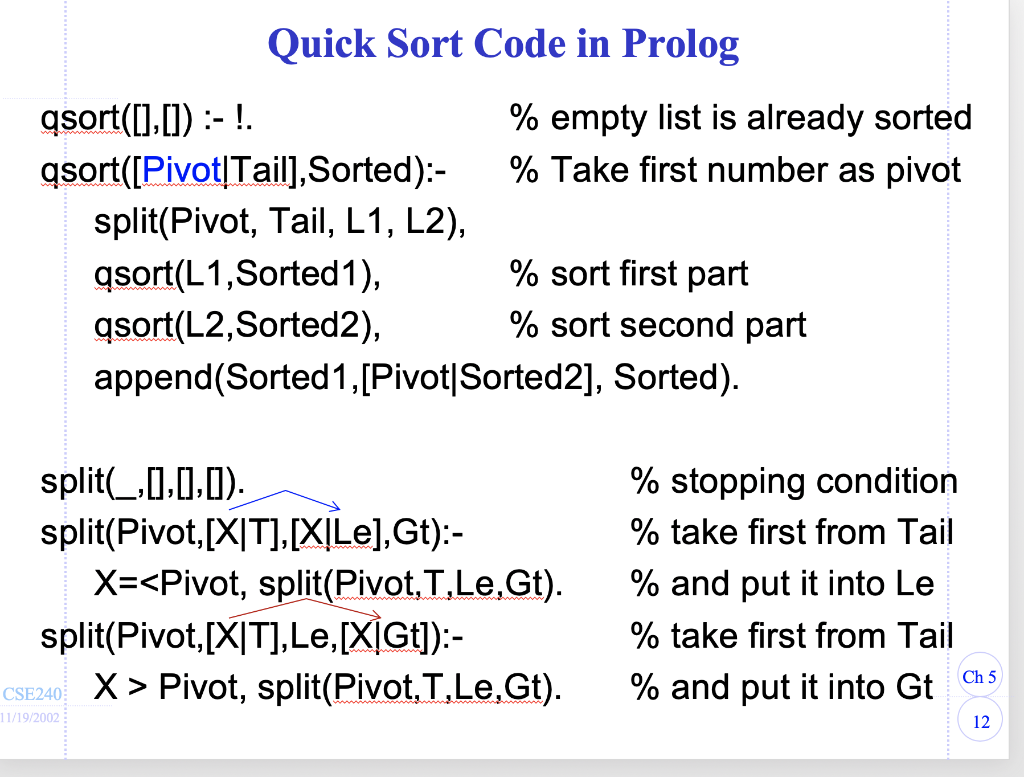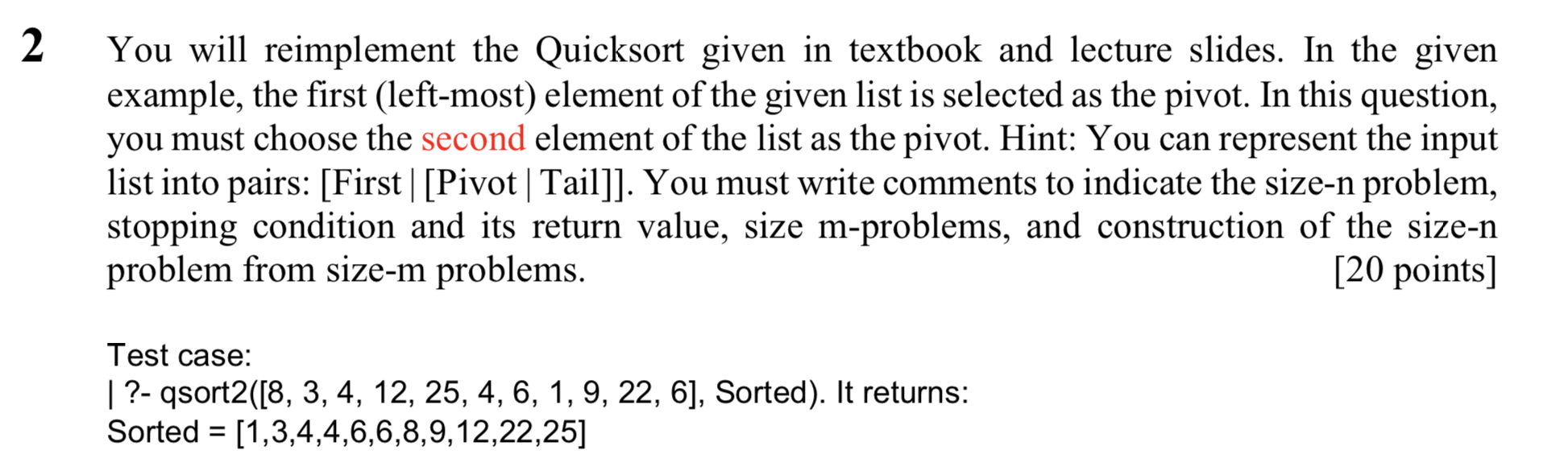# (Solved) : Reimplement Quicksort Given Textbook Lecture Slides Given Example First Left Element Given Q42783858 . . .1. You will reimplement the Quicksort given in textbook and lectureslides. In the given example, the first (left-most) element of thegiven list is selected as the pivot. In this question, you mustchoose the second element of the list as the pivot. Hint: You canrepresent the input list into pairs: [First | [Pivot | Tail]]. Youmust write comments to indicate the size-n problem, stoppingcondition and its return value, size m-problems, and constructionof the size-n problem from size-m problems.

Quick Sort Code in Prolog asort([],[]) :- ! % empty list is already sorted asort([Pivot[Tail], Sorted):- % Take first number as pivot split(Pivot, Tail, L1, L2), asort(L1, Sorted1), % sort first part qsort(L2, Sorted2), % sort second part append(Sorted1,[Pivot|Sorted2], Sorted). split(_,[],[],[]). split(Pivot,[X|T],[X|Le], Gt):- X=<Pivot, split(Pivot, T,Le, Gt). split(Pivot, [X|T], Le, [X[Gt]):- CSE240 X > Pivot, split(Pivot.T.Le, Gt). % stopping condition % take first from Tail % and put it into Le % take first from Tail % and put it into Gtchs 11/19/2002 You will reimplement the Quicksort given in textbook and lecture slides. In the given example, the first (left-most) element of the given list is selected as the pivot. In this question, you must choose the second element of the list as the pivot. Hint: You can represent the input list into pairs: [First | [Pivot | Tail]]. You must write comments to indicate the size-n problem, stopping condition and its return value, size m-problems, and construction of the size-n problem from size-m problems. [20 points] Test case: | ?- qsort2([8, 3, 4, 12, 25, 4, 6, 1, 9, 22, 6], Sorted). It returns: Sorted = [1,3,4,4,6,6,8,9,12,22,25] Show transcribed image text Quick Sort Code in Prolog asort([],[]) :- ! % empty list is already sorted asort([Pivot[Tail], Sorted):- % Take first number as pivot split(Pivot, Tail, L1, L2), asort(L1, Sorted1), % sort first part qsort(L2, Sorted2), % sort second part append(Sorted1,[Pivot|Sorted2], Sorted). split(_,[],[],[]). split(Pivot,[X|T],[X|Le], Gt):- X= Pivot, split(Pivot.T.Le, Gt). % stopping condition % take first from Tail % and put it into Le % take first from Tail % and put it into Gtchs 11/19/2002
You will reimplement the Quicksort given in textbook and lecture slides. In the given example, the first (left-most) element of the given list is selected as the pivot. In this question, you must choose the second element of the list as the pivot. Hint: You can represent the input list into pairs: [First | [Pivot | Tail]]. You must write comments to indicate the size-n problem, stopping condition and its return value, size m-problems, and construction of the size-n problem from size-m problems. [20 points] Test case: | ?- qsort2([8, 3, 4, 12, 25, 4, 6, 1, 9, 22, 6], Sorted). It returns: Sorted = [1,3,4,4,6,6,8,9,12,22,25]

Answer to You will reimplement the Quicksort given in textbook and lecture slides. In the given example, the first (left-most) ele…

We are the best freelance writing portal. Looking for online writing, editing or proofreading jobs? We have plenty of writing assignments to handle.